Next: Models for Galactic sources Up: Bayesian model comparison applied Previous: Why not to use

# The Bayesian way out: how to use of experimental data to update the credibility of hypotheses

We think that the solution to the above problems consists in changing radically our attitude, instead of seeking for new prescriptions' which might cure a trouble but generate others. The so called Bayesian approach, based on the natural idea of probability as degree of belief' and on the rules of logic, seems to us to be the proper way to deal with our problem. A key role in this approach is played by Bayes' theorem, which, apart from normalization constant, can be stated as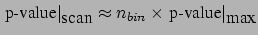Data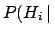Data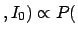(4)

where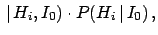stand the hypotheses that could produce the Data with likelihood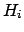Data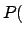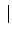.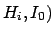Dataand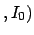are, respectively, the posterior and prior probabilities, i.e. with or without taking into account the information provided by the Data.stands for the general status of information, which is usually considered implicit and will then be omitted in the following formulae.

The presence of priors, considered a weak point by opposer's of the Bayesian theory, is one of the points of force of the theory. First, because priors are necessary to make the probability inversion' of Eq. (4). Second, because in this approach all relevant conditions must be clearly stated, instead of being hidden in the method or left to the arbitrariness of the practitioner. Third, because prior knowledge can be properly incorporated in the analysis to integrate missing or deteriorated experimental information (and whatever it is done should be stated explicitly!). Finally, because the clear separation of prior and likelihood in Eq. (4) allows to publish the results in a way independent from, if the priors might differ largely within the members of the scientific community. In particular, the Bayes factor, defined as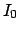(5)

is the factor which changes the bet odds' (i.e. probability ratios) in the light of the new data. In fact, dividing member to member Eq. (4) written for hypothesesand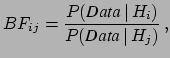, we get

 posterior odds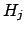prior odds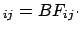(6)

Since we shall speak later about models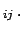, the odd ratio updating is given by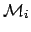(7)

Some general remarks are in order.
• Conclusions depend only on the observed data and on the previous knowledge. In particular they do not depend on unobserved data which are rarer than the data really observed (that is what p-values imply).
• At least two models have to be taken into account, and the likelihood for each model must be specified.
• There is no need to consider all possible models', since what matters are relative beliefs.
• Similarly, there is no need that the model must be declared before the data are taken, or analyzed. What matters is that the initial beliefs should be based on general arguments about the plausibility of each model and on agreement with other experimental information, other than Data.
An analogue of Eq. (4) applies to the parameters of a model. For example, if, given a model, we are interested to the rate of g.w. on Earth,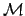, Bayes' theorem gives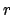(8)

where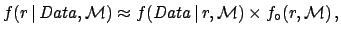stand for probability density functions (pdf) Also in this case, a prior independent way of reporting the result is possible. The difficulty of dealing with an infinite number of Bayes factors (precisely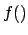, given each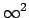and) can be overcome defining a function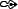ofwhich gives the Bayes factor with respect to a reference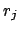. This function is particularly useful ifis chosen to be the asymptotic value at which the experiment looses completely sensitivity. For g.w. search this asymptotic value is simply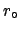. In other cases it could be an infinite particle mass  or an infinite mass scale . In the case of g.w. rate, extensively discussed in Ref. , we get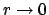(9)

where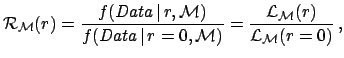is the model dependent likelihood. [Note that, indeed, in the limit ofthe likelihood depends only on the background expectation and not on the specific model. Therefore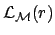, where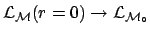stands for the model background alone''.] Thisfunction has the meaning of relative belief updating factor , since it tells us how we must modify our beliefs of the different values of, given the observed data. In the region wherevanishes, the corresponding values ofare excluded. On the other hand, in the region whereis about unity, the data are unable to change our beliefs, i.e. we have lost sensitivity. The region of transition between 0 and 1 defines the sensitivity bound, a concept that does not have a probabilistic meaning and, since it does not refers to terms such as confidence', does not cause the typical misinterpretations of the frequentistic `confidence upper/lower limits' (for a recent example of results using these ideas see Ref. ). Values ofpreferred by the data are spotted by large value of. We shall in the sequel how a plot of thefunction gives an immediate representation of what the data tell about a parameter (Figs. 3 and 4). Another interesting feature of this function is that, if several independent data sets are available, each providing some information about model, the global information is obtained multiplying the variousfunctions: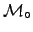All data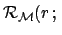Data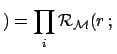(10)

SubsectionsNext: Models for Galactic sources Up: Bayesian model comparison applied Previous: Why not to use
Giulio D'Agostini 2005-01-09